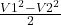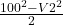## Water vapor enters an insulated nozzle operating at steady state with a velocity of 100 m/s and specific enthalpy of 3445.3 kJ/kg, and exits

Question

Water vapor enters an insulated nozzle operating at steady state with a velocity of 100 m/s and specific enthalpy of 3445.3 kJ/kg, and exits with specific enthalpy of 3051.1 kJ/kg. The velocity at the exit is most closely

(a) 104 m/s
(b) 636 m/s
(c) 888 m/s
(d) 894 m/s

in progress 0
6 months 2021-07-28T05:22:11+00:00 1 Answers 36 views 0

(a) 104 m/s

Explanation:

enters with specific enthalpy  ‘h1’ = 3445.3 kJ/kg

exits with specific enthalpy ‘h2’ 3051.1 kJ/kg

velocity ‘V1’= 100 m/s

In order to find velocity at the exit ‘V2’, we use this relation

0 = Qcv – Wcv + m[(h1-h2) ++ g(z1-z2)]

0= [Qcv + m[(h1-h2) +]

0=( h1-h2) + ()

0= (3445.3-3051.1)+0= 394.2 +-394.2=-788.4 = 10000-V2²

V2²= 10000+ 788.4

V2²= 10784.44

Taking square root on both sides

V2=103.84

V2≈ 104m/s

Therefore, The velocity at the exit is most closely  104m/s